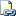# | (Bitwise OR) (Transact-SQL)

Performs a bitwise logical OR operation between two specified integer values as translated to binary expressions within Transact-SQL statements.Transact-SQL Syntax Conventions

## Syntax

``````expression | expression
``````

## Arguments

• expression
Is any valid expression of the integer data type category, or the bit, binary, or varbinary data types. expression is treated as a binary number for the bitwise operation.

Note

Only one expression can be of either binary or varbinary data type in a bitwise operation.

## Remarks

The bitwise | operator performs a bitwise logical OR between the two expressions, taking each corresponding bit for both expressions. The bits in the result are set to 1 if either or both bits (for the current bit being resolved) in the input expressions have a value of 1; if neither bit in the input expressions is 1, the bit in the result is set to 0.

If the left and right expressions have different integer data types (for example, the left expression is smallint and the right expression is int), the argument of the smaller data type is converted to the larger data type. In this example, the smallintexpression is converted to an int.

## Result Types

Returns an int if the input values are int, a smallint if the input values are smallint, or a tinyint if the input values are tinyint.

## Examples

The following example creates a table with int data types to show the original values and puts the table into one row.

``````USE tempdb;
GO
IF EXISTS (SELECT * FROM INFORMATION_SCHEMA.TABLES
WHERE TABLE_NAME = 'bitwise')
DROP TABLE bitwise;
GO
CREATE TABLE bitwise
(
a_int_value int NOT NULL,
b_int_value int NOT NULL
);
GO
INSERT bitwise VALUES (170, 75);
GO
``````

The following query performs the bitwise OR on the a_int_value and b_int_value columns.

``````USE tempdb;
GO
SELECT a_int_value | b_int_value
FROM bitwise;
GO
``````

Here is the result set.

``````-----------
235

(1 row(s) affected)
``````

The binary representation of 170 (a_int_value or `A`, below) is `0000 0000 1010 1010`. The binary representation of 75 (b_int_value or `B`, below) is `0000 0000 0100 1011`. Performing the bitwise OR operation on these two values produces the binary result `0000 0000 1110 1011`, which is decimal 235.

``````(A | B)
0000 0000 1010 1010
0000 0000 0100 1011
-------------------
0000 0000 1110 1011
``````